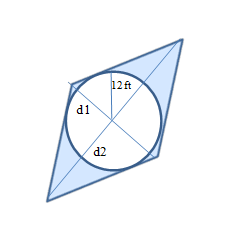Chapter 8.4, Problem 25E### Elementary Geometry for College St...

6th Edition
Daniel C. Alexander + 1 other
ISBN: 9781285195698

#### Solutions

Chapter
Section### Elementary Geometry for College St...

6th Edition
Daniel C. Alexander + 1 other
ISBN: 9781285195698
Textbook Problem
19 views

# In exercises 23-26, find the exact areas of shaded region.d1=30 ftd2=40 ftRhombus

To determine

To Find:

The areas of the shaded region of the rhombus.

Explanation

To find the area of the shaded region of the rhombus, find the area of the rhombus and circle and then subtract the area of the circle from the area of the rhombus.

It is given that the diagonals of the rhombus are d1=30ftandd2=40ft.

Therefore, the area of the rhombus is A=12d1d2.

A=123040=600.

The area of the rhombus is 600 ft2

### Still sussing out bartleby?

Check out a sample textbook solution.

See a sample solution

#### The Solution to Your Study Problems

Bartleby provides explanations to thousands of textbook problems written by our experts, many with advanced degrees!

Get Started

#### In Problems 35-42, simplify each complex fraction. 41.

Mathematical Applications for the Management, Life, and Social Sciences

#### Determine the infinite limit. limx2+x22x8x25x+6

Single Variable Calculus: Early Transcendentals, Volume I

#### Place the following set of n = 20 scores in a frequency distribution table.

Essentials of Statistics for The Behavioral Sciences (MindTap Course List)

#### Convert the expressions in Exercises 6584 to power form. x23

Finite Mathematics and Applied Calculus (MindTap Course List)

#### sinx=ex+ex2

Study Guide for Stewart's Single Variable Calculus: Early Transcendentals, 8th